Mathematics

34 affiliated resources

Search Resources

View
Selected filters: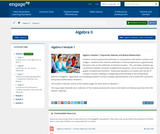Conditional Remix & Share Permitted
CC BY-NC-SA
Rating

Students connect polynomial arithmetic to computations with whole numbers and integers.  Students learn that the arithmetic of rational expressions is governed by the same rules as the arithmetic of rational numbers.  This unit helps students see connections between solutions to polynomial equations, zeros of polynomials, and graphs of polynomial functions.  Polynomial equations are solved over the set of complex numbers, leading to a beginning understanding of the fundamental theorem of algebra.  Application and modeling problems connect multiple representations and include both real world and purely mathematical situations.

Subject:
Algebra
Material Type:
Module
Provider:
New York State Education Department
Provider Set:
EngageNY
05/14/2013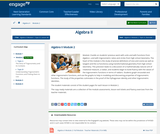Conditional Remix & Share Permitted
CC BY-NC-SA
Rating

Module 2 builds on students previous work with units and with functions from Algebra I, and with trigonometric ratios and circles from high school Geometry. The heart of the module is the study of precise definitions of sine and cosine (as well as tangent and the co-functions) using transformational geometry from high school Geometry. This precision leads to a discussion of a mathematically natural unit of rotational measure, a radian, and students begin to build fluency with the values of the trigonometric functions in terms of radians. Students graph sinusoidal and other trigonometric functions, and use the graphs to help in modeling and discovering properties of trigonometric functions. The study of the properties culminates in the proof of the Pythagorean identity and other trigonometric identities.

Subject:
Algebra
Material Type:
Module
Provider:
New York State Education Department
Provider Set:
EngageNY
08/15/2014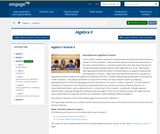Conditional Remix & Share Permitted
CC BY-NC-SA
Rating

In this module, students synthesize and generalize what they have learned about a variety of function families.  They extend the domain of exponential functions to the entire real line (N-RN.A.1) and then extend their work with these functions to include solving exponential equations with logarithms (F-LE.A.4).  They explore (with appropriate tools) the effects of transformations on graphs of exponential and logarithmic functions.  They notice that the transformations on a graph of a logarithmic function relate to the logarithmic properties (F-BF.B.3).  Students identify appropriate types of functions to model a situation.  They adjust parameters to improve the model, and they compare models by analyzing appropriateness of fit and making judgments about the domain over which a model is a good fit.  The description of modeling as, the process of choosing and using mathematics and statistics to analyze empirical situations, to understand them better, and to make decisions, is at the heart of this module.  In particular, through repeated opportunities in working through the modeling cycle (see page 61 of the CCLS), students acquire the insight that the same mathematical or statistical structure can sometimes model seemingly different situations.

Subject:
Algebra
Material Type:
Module
Provider:
New York State Education Department
Provider Set:
EngageNY
09/16/2014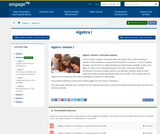Conditional Remix & Share Permitted
CC BY-NC-SA
Rating

In this module, students reconnect with and deepen their understanding of statistics and probability concepts first introduced in Grades 6, 7, and 8. Students develop a set of tools for understanding and interpreting variability in data, and begin to make more informed decisions from data. They work with data distributions of various shapes, centers, and spreads. Students build on their experience with bivariate quantitative data from Grade 8. This module sets the stage for more extensive work with sampling and inference in later grades.

Subject:
Statistics and Probability
Material Type:
Module
Provider:
New York State Education Department
Provider Set:
EngageNY
08/01/2013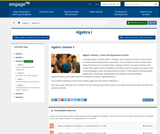Conditional Remix & Share Permitted
CC BY-NC-SA
Rating

In earlier grades, students define, evaluate, and compare functions and use them to model relationships between quantities. In this module, students extend their study of functions to include function notation and the concepts of domain and range. They explore many examples of functions and their graphs, focusing on the contrast between linear and exponential functions. They interpret functions given graphically, numerically, symbolically, and verbally; translate between representations; and understand the limitations of various representations.

Subject:
Algebra
Material Type:
Module
Provider:
New York State Education Department
Provider Set:
EngageNY
09/17/2013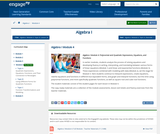Conditional Remix & Share Permitted
CC BY-NC-SA
Rating

In earlier modules, students analyze the process of solving equations and developing fluency in writing, interpreting, and translating between various forms of linear equations (Module 1) and linear and exponential functions (Module 3). These experiences combined with modeling with data (Module 2), set the stage for Module 4. Here students continue to interpret expressions, create equations, rewrite equations and functions in different but equivalent forms, and graph and interpret functions, but this time using polynomial functions, and more specifically quadratic functions, as well as square root and cube root functions.

Subject:
Algebra
Material Type:
Module
Provider:
New York State Education Department
Provider Set:
EngageNY
09/17/2013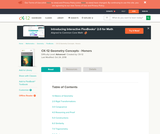Conditional Remix & Share Permitted
CC BY-NC
Rating

This Geometry Concept Collection is a rigorous presentation of high school geometry. It is fully correlated with the Common Core State Standards.

Subject:
Geometry
Material Type:
Full Course
Homework/Assignment
Interactive
Lecture Notes
Lesson Plan
Textbook
Unit of Study
Provider:
CK-12 Foundation
Author:
Kaitlyn Spong
11/18/2020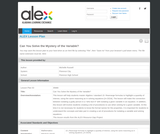Some Rights Reserved
Rating

This lesson will help students master Algebra I standard 15: Rearrange formulas to highlight a quantity of interest, using the same reasoning as in solving equations [A-CED4]. The lesson will make the connection between isolating a guilty person in a "who-dun-it" with isolating a given variable in an equation. In addition, this lesson will involve students creating a list of procedures to use when solving for a given variable. At this time it is not necessary for students to know the formal names for the properties. It is important for students to understand the concepts and take part in creating a set of procedures for isolating a variable and solving equations. This lesson results from the ALEX Resource Gap Project.

Subject:
Mathematics
Material Type:
Lesson Plan
Provider:
Alabama Learning Exchange (ALEX)
02/10/2021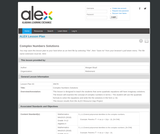Some Rights Reserved
Rating

This lesson is designed to teach the students that some quadratic equations will have imaginary solutions. The lesson will examine the concept of complex numbers in terms i. The student will use the quadratic formula to solve the equations and write the the solutions in the form a +bi. This lesson results from the ALEX Resource Gap Project.

Subject:
Mathematics
Material Type:
Lesson Plan
Provider:
Alabama Learning Exchange (ALEX)
02/10/2021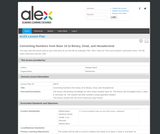Some Rights Reserved
Rating

The lesson will develop knowledge for other bases besides base 10. The lesson will investigate base 2, base 8, and base 16. The student will write numbers using expanded notation. This lesson results from the ALEX Resource Gap Project.

Subject:
Mathematics
Material Type:
Lesson Plan
Provider:
Alabama Learning Exchange (ALEX)
02/10/2021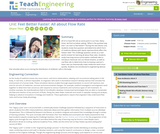Educational Use
Rating

All of us have felt sick at some point in our lives. Many times, we find ourselves asking, "What is the quickest way that I can start to feel better?" During this two-lesson unit, students study that question and determine which form of medicine delivery (pill, liquid, injection/shot) offers the fastest relief. This challenge question serves as a real-world context for learning all about flow rates. Students study how long various prescription methods take to introduce chemicals into our blood streams, as well as use flow rate to determine how increasing a person's heart rate can theoretically make medicines work more quickly. Students are introduced to engineering devices that simulate what occurs during the distribution of antibiotic cells in the body.

Subject:
Mathematics
Engineering
Life Science
Physics
Material Type:
Unit of Study
Provider:
TeachEngineering
Provider Set:
TeachEngineering
Author:
Michelle Woods
09/18/2014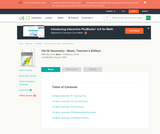Conditional Remix & Share Permitted
CC BY-NC-SA
Rating

The Teacher's Edition covers tips, common errors, enrichment, differentiated instruction and problem solving for teaching CK-12's Basic Geometry Student Edition. The solution and assessment guides are available upon request.

Subject:
Geometry
Material Type:
Textbook
Provider:
CK-12 Foundation
Provider Set:
CK-12 FlexBook
Author:
Dirga, Kathryn
Jordan, Lori
08/24/2011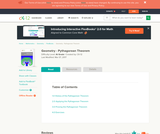Conditional Remix & Share Permitted
CC BY-NC-SA
Rating

This stand-alone module examines the history, applications, and various proofs of the Pythagorean Theorem. The module also includes student activities and exercise problems. The module assumes the reader has a basic geometry background.

Subject:
Geometry
Material Type:
Textbook
Provider:
CK-12 Foundation
Provider Set:
CK-12 FlexBook
Author:
Joy (Editor)
Laura
Sheng
Swenson
11/08/2010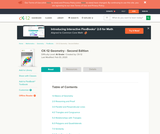Conditional Remix & Share Permitted
CC BY-NC-SA
Rating

CK-12's Geometry - Second Edition is a clear presentation of the essentials of geometry for the high school student. Topics include: Proofs, Triangles, Quadrilaterals, Similarity, Perimeter & Area, Volume, and Transformations.

Subject:
Geometry
Material Type:
Textbook
Provider:
CK-12 Foundation
Provider Set:
CK-12 FlexBook
Author:
Dirga, Kathryn
Jordan, Lori
11/16/2010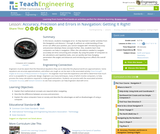Educational Use
Rating

In this lesson, students will investigate error. As shown in earlier activities from navigation lessons 1 through 3, without an understanding of how errors can affect your position, you cannot navigate well. Introducing accuracy and precision will develop these concepts further. Also, students will learn how computers can help in navigation. Often, the calculations needed to navigate accurately are time consuming and complex. By using the power of computers to do calculations and repetitive tasks, one can quickly see how changing parameters likes angles and distances and introducing errors will affect their overall result.

Subject:
Mathematics
Geometry
Trigonometry
Engineering
Material Type:
Activity/Lab
Lesson Plan
Provider:
TeachEngineering
Provider Set:
TeachEngineering
Author:
Janet Yowell
Jeff White
Malinda Schaefer Zarske
Matt Lippis
09/18/2014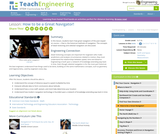Educational Use
Rating

In this lesson, students will learn how great navigators of the past stayed on course that is, the historical methods of navigation. The concepts of dead reckoning and celestial navigation are discussed.

Subject:
Mathematics
Engineering
Material Type:
Activity/Lab
Lesson Plan
Provider:
TeachEngineering
Provider Set:
TeachEngineering
Author:
Janet Yowell
Jeff White
Malinda Schaefer Zarske
09/18/2014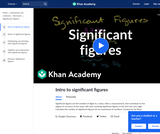Conditional Remix & Share Permitted
CC BY-NC-SA
Rating

Significant figures are the number of digits in a value, often a measurement, that contribute to the degree of accuracy of the value. We start counting significant figures at the first non-zero digit. Calculate the number of significant figures for an assortment of numbers.

Subject:
Mathematics
Material Type:
Lesson
Provider:
Provider Set:
Author:
Salman Khan
11/17/2020Educational Use
Rating

After their carts collide in a hardware store, two teachers discover that they both bought the same items in different quantities. With limited information, this segment demonstrates how to use an equation to determine the cost of each item.

Subject:
Mathematics
Algebra
Material Type:
Lesson
Provider:
PBS LearningMedia
Author:
New York Network
WNET
05/13/2010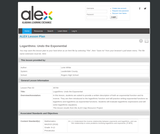Some Rights Reserved
Rating

In this lesson, students are asked to provide a written description of both an exponential function and its inverse. They are then introduced to the logarithmic function and will practice writing exponential functions as logarithms and logarithms as exponential functions. Students will evaluate logarithmic expressions and will solve logarithmic equations. This lesson results from the ALEX Gap Resource Project.

Subject:
Mathematics
Material Type:
Lesson Plan
Provider:
Alabama Learning Exchange (ALEX)
02/10/2021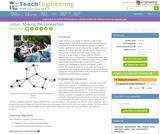Educational Use
Rating

Graph theory is a visual way to represent relationships between objects. One of the simplest uses of graph theory is a family tree that shows how different people are related. Another application is social networks like Facebook, where a network of "friends" and their "friends" can be represented using graphs. Students learn and apply concepts and methods of graph theory to analyze data for different relationships such as friendships and physical proximity. They are asked about relationships between people and how those relationships can be illustrated. As part of the lesson, students are challenged to find the social graph of their friends. This prepares students for the associated activity during which they simulate and analyze the spread of disease using graph theory by assuming close proximity to an infected individual causes the disease to spread.

Subject:
Mathematics
Engineering
Material Type:
Lesson Plan
Provider:
TeachEngineering
Provider Set:
TeachEngineering
Author:
Brian Sandall
Steve Hamersky
09/18/2014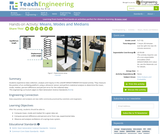Educational Use
Rating

Students experience data collection, analysis and inquiry in this LEGO® MINDSTORMS® NXT -based activity. They measure the position of an oscillating platform using a ultrasonic sensor and perform statistical analysis to determine the mean, mode, median, percent difference and percent error for the collected data.

Subject:
Mathematics
Engineering
Physics
Material Type:
Activity/Lab
Provider:
TeachEngineering
Provider Set:
TeachEngineering
Author:
Irina Igel
Noam Pillischer
Ronald Poveda
09/18/2014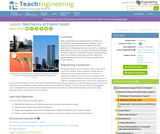Educational Use
Rating

After conducting the associated activity, students are introduced to the material behavior of elastic solids. Engineering stress and strain are defined and their importance in designing devices and systems is explained. How engineers measure, calculate and interpret properties of elastic materials is addressed. Students calculate stress, strain and modulus of elasticity, and learn about the typical engineering stress-strain diagram (graph) of an elastic material.

Subject:
Mathematics
Engineering
Life Science
Physics
Material Type:
Lesson Plan
Provider:
TeachEngineering
Provider Set:
TeachEngineering
Author:
Brandi N. Briggs
Marissa H. Forbes
09/18/2014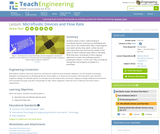Educational Use
Rating

Students obtain a basic understanding of microfluidic devices, how they are developed and their uses in the medical field. After conducting the associated activity, they watch a video clip and learn about flow rate and how this relates to the speed at which medicine takes effect in the body. What they learn contributes to their ongoing objective to answer the challenge question presented in lesson 1 of this unit. They conclude by solving flow rate problems provided on a worksheet.

Subject:
Mathematics
Algebra
Engineering
Life Science
Physics
Material Type:
Lesson Plan
Provider:
TeachEngineering
Provider Set:
TeachEngineering
Author:
Michelle Woods
09/18/2014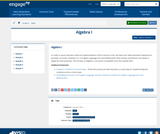Conditional Remix & Share Permitted
CC BY-NC-SA
Rating

In order to assist educators with the implementation of the Common Core, the New York State Education Department provides curricular modules in P-12 English Language Arts and Mathematics that schools and districts can adopt or adapt for local purposes. The full year of Algebra I curriculum is available below.

Subject:
Mathematics
Algebra
Material Type:
Full Course
Provider:
New York State Education Department
Provider Set:
EngageNY
10/15/2014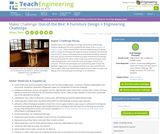Educational Use
Rating

Student teams are challenged to design and build architecturally inspired cardboard furniture, guided by the steps of the engineering design process. They cultivate their industrial engineering and design skills to design furnishings that meet functional, aesthetic and financial requirements. Given constraints that include limited building materials and tools, groups research architectural styles and period furnishings. The teams brainstorm ideas, make small-scale quick prototypes, then make detailed plans and create full-scale prototypes of their best solutions. The full-size prototypes are evaluated by peer critique for aesthetic alignment to the targeted architectural style and tested for functionality. After final refinements, teams present their concepts and display their final prototype furnishings in an exhibition.

Subject:
Measurement and Data
Engineering
Material Type:
Activity/Lab
Provider:
TeachEngineering
Provider Set:
MakerChallenges
Author:
Jenny Montgomery
Marta Wojcik
07/14/2017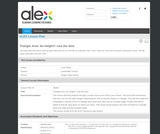Some Rights Reserved
Rating

This lesson will lead students through a review of the proof of the Law of Sines. This proof will remind them that they can use the right triangle relationship for Sine to find the height of a triangle. They will then apply this knowledge to find the area of a triangle when given two sides and an included angle. Finally, they will be asked to find the area when no values are given. This result should produce the Area Formula for a triangle given two sides and the included angle. This lesson results from the ALEX Resource Gap Project.

Subject:
Mathematics
Material Type:
Lesson Plan
Provider:
Alabama Learning Exchange (ALEX)
02/10/2021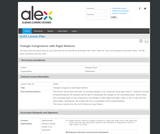Some Rights Reserved
Rating

This lesson will provide instruction on proving triangles to be congruent using rigid motions. Using the concept of transformations, the students will be able to manipulate the triangle on the coordinate plane. When using the coordinate plane to test congruence, the triangle or other object will slide, rotate, or flip to map onto the other object. Sometimes, the student will use a combination of the transformations. This lesson results from the ALEX Resource Gap Project.

Subject:
Mathematics
Material Type:
Lesson Plan
Provider:
Alabama Learning Exchange (ALEX)
02/10/2021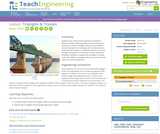Educational Use
Rating

Students learn about the fundamental strength of different shapes, illustrating why structural engineers continue to use the triangle as the structural shape of choice. Examples from everyday life are introduced to show how this shape is consistently used for structural strength. Along with its associated activity, this lesson empowers students to explore the strength of trusses made with different triangular elements to evaluate the various structural properties.

Subject:
Mathematics
Engineering
Material Type:
Lesson Plan
Provider:
TeachEngineering
Provider Set:
TeachEngineering
Author:
Amanda Guiliani
Darcie Chinnis
Malinda Schaefer Zarske
Scott Duckworth
09/18/2014Educational Use
Rating

This video segment from Regents Review 2.0 uses a trigonometry equation to determine the height of a flagpole located in front of the school.

Subject:
Mathematics
Material Type:
Lesson
Provider:
PBS LearningMedia
Author:
New York Network
WNET
05/13/2010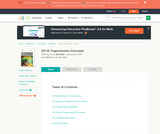Conditional Remix & Share Permitted
CC BY-NC
Rating

CK-12 Foundation's Trigonometry FlexBook is an introduction to trigonometry for the high school student. It includes chapters on graphs of trigonometric functions, trigonometric identities, inverse trigonometric functions, triangles and vectors, and the polar system.

Subject:
Trigonometry
Material Type:
Textbook
Provider:
CK-12 Foundation
Provider Set:
CK-12 FlexBook
11/18/2020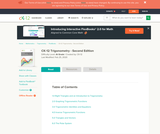Conditional Remix & Share Permitted
CC BY-NC-SA
Rating

CK-12 Foundation's Trigonometry FlexBook is an introduction to trigonometry for the high school student. Topics include: Trigonometric Identities & Equations, Circular Functions, and Polar Equations & Complex Numbers.

Subject:
Trigonometry
Material Type:
Textbook
Provider:
CK-12 Foundation
Provider Set:
CK-12 FlexBook
Author:
Fortgang, Art
Hayes, Andrea
Jordan, Lori
Landers, Mara
Meery, Brenda
Ottman, Larry
08/12/2010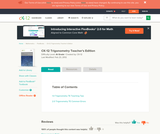Conditional Remix & Share Permitted
CC BY-NC-SA
Rating

CK-12 Trigonometry Teacher's Edition provides tips and common errors for teaching CK-12 Trigonometry Student Edition. The solution and assessment guides are available upon request.

Subject:
Trigonometry
Material Type:
Textbook
Provider:
CK-12 Foundation
Provider Set:
CK-12 FlexBook
Author:
Rawley, Eve
06/25/2011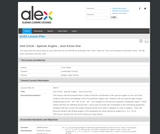Some Rights Reserved
Rating

This lesson will demonstrate that in order to find the coordinates of the special angles on the unit circle, students will need a knowledge of the first quadrant angles only. Students will use special right triangle relationships for 30degrees - 60degrees -90degrees or 45degrees - 45degrees - 90degrees triangles to find the first quadrant coordinate values. These values will then be reflected across the x- and y-axis to locate the coordinates in the remaining quadrants. Students will also convert the angle measurements from units in degrees to units in radians. They will become familiar with finding angles in the quadrants by using reference angles (&pi;-x, &pi;+x. 2&pi;-x). This lesson results from the ALEX Resource Gap Project.

Subject:
Mathematics
Material Type:
Lesson Plan
Provider:
Alabama Learning Exchange (ALEX)
02/10/2021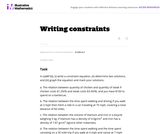Unrestricted Use
CC BY
Rating

The purpose of this task is to give students practice writing a constraint equation for a given context. Instruction accompanying this task should introduce the notion of a constraint equation as an equation governing the possible values of the variables in question.

Subject:
Mathematics
Algebra
Material Type:
Activity/Lab
Provider:
Illustrative Mathematics
Provider Set:
Illustrative Mathematics
Author:
Illustrative Mathematics